Courses

# Cross Product of Vectors Mechanical Engineering Notes | EduRev

## Mechanical Engineering : Cross Product of Vectors Mechanical Engineering Notes | EduRev

The document Cross Product of Vectors Mechanical Engineering Notes | EduRev is a part of the Mechanical Engineering Course Engineering Mechanics - Notes, Videos, MCQs & PPTs.
All you need of Mechanical Engineering at this link: Mechanical Engineering

Cross Product

The cross product of two vectors a andb is defined only in three-dimensional space and is denoted by a × b. In Physics, sometimes the notation ab is used, though this is avoided in mathematics to avoid confusion with the Exterior Product.

The cross product a × b is defined as a vector c that is Perpendicular (orthogonal) to both a andb, with a direction given by the Right-Hand Rule and a magnitude equal to the area of the Parallelogram that the vectors span.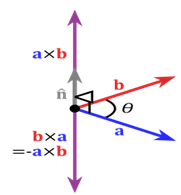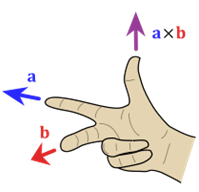The cross product is defined by the formula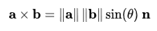where θ is the Angle between a and b in the plane containing them (hence, it is between 0° and 180°), ‖a‖ and ‖b‖ are the Magnitudes of vectors a and b, and n is a Unit Vector perpendicular to the plane containing a and b in the direction given by the right-hand rule (illustrated). If the vectors a andb are parallel (i.e., the angle θ between them is either 0° or 180°), by the above formula, the cross product of a and b is the Zero Vector 0.

By convention, the direction of the vector n is given by the right-hand rule, where one simply points the forefinger of the right hand in the direction of a and the middle finger in the direction of b. Then, the vector n is coming out of the thumb (see the picture on the right). Using this rule implies that the cross-product is Anti-Commutative, i.e., b * a = −(a * b). By pointing the forefinger toward b first, and then pointing the middle finger toward a, the thumb will be forced in the opposite direction, reversing the sign of the product vector.

Using the cross product requires the handedness of the coordinate system to be taken into account (as explicit in the definition above). If a Left-Handed Coordinate System is used, the direction of the vector n is given by the left-hand rule and points in the opposite direction.

This, however, creates a problem because transforming from one arbitrary reference system to another (e.g., a mirror image transformation from a right-handed to a left-handed coordinate system), should not change the direction of n. The problem is clarified by realizing that the cross product of two vectors is not a (true) vector, but rather a Pseudovector. See Cross Product and Handedness for more detail.

In some two-dimensional and many of the three-dimensional problems to follow, it is convenient to use a vector approach for moment calculations. The moment of F about point A of Fig. 2/8b may be represented by the cross-product expression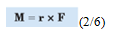Where r is a position vector which runs from the moment reference point A to any point on the line of action of F. The magnitude of this expression is given by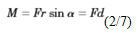which agrees with the moment magnitude as given by Eq. 2/5. Note that the moment arm  d =r sin a does not depend on the particular point on the line of action of F to which the vector r is directed. We establish the direction and sense of M by applying the right-hand rule to the sequence r*F. If the ﬁngers of the right hand are curled in the direction of rotation from the positive sense of r to the positive sense of F, then the thumb points in the positive sense of M. We must maintain the sequence r*F, because the sequence F*r would produce a vector with a sense opposite to that of the correct moment. As was the case with the scalar approach, the moment M may be thought of as the moment about point A or as the moment about the line O-O which passes through point A and is perpendicular to the plane containing the vectors r and F. When we evaluate the moment of a force about a given point, the choice between using the vector cross product or the scalar expression depends on how the geometry of the problem is specified. If we know or can easily determine the perpendicular distance between the line of action of the force and the moment center, then the scalar approach is generally simpler. If, however, F and r are not perpendicular and are easily expressible in vector notation, then the cross-product expression is often preferable.

Offer running on EduRev: Apply code STAYHOME200 to get INR 200 off on our premium plan EduRev Infinity!

## Engineering Mechanics - Notes, Videos, MCQs & PPTs

30 videos|72 docs|65 tests

,

,

,

,

,

,

,

,

,

,

,

,

,

,

,

,

,

,

,

,

,

;